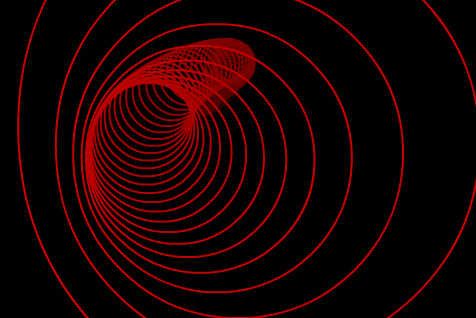Categories

# Smooth circles in Papervision

Here’s a handy little class that will make a smooth 3D circle out of curved lines in Papervision3D. Add an nice little sine wave movement curve and you have this! Click on the image to see it in action.Here’s the source :

Circle class :

```
// Author : Seb Lee-Delisle
// Blog : www.sebleedelisle.com
// Company : www.pluginmedia.net
// Mail : sebleedelisle@gmail.com
//

// Full details at

package net.pluginmedia.pv3d
{
import org.papervision3d.core.geom.Lines3D;
import org.papervision3d.core.geom.renderables.Line3D;
import org.papervision3d.core.geom.renderables.Vertex3D;
import org.papervision3d.core.math.Number3D;
import org.papervision3d.materials.special.LineMaterial;

public class Circle3D extends Lines3D
{
public function Circle3D(mat:LineMaterial, radius : Number=100, divisions : int = 8, lineWeight : Number = 2, startAngle : Number = 0, endAngle : Number = 360)
{
super(mat);
}

public function addCircle(x:Number, y:Number, z:Number, r:Number, d:Number = 8, startAngle:Number =0, endAngle:Number = 360, linethickness:Number = 2):void
{
var temp : Number3D = new Number3D(r,0,0);
var tempcurve:Number3D = new Number3D(0,0,0);
var joinends : Boolean;
var i:int;
var pointcount : int;

var angle:Number = (endAngle-startAngle)/d;
var curveangle : Number = angle/2;

tempcurve.rotateY(curveangle+startAngle);

if(endAngle-startAngle<360)
{
joinends = false;
pointcount = d+1;
}
else
{
joinends = true;
pointcount = d;
}

temp.rotateY(startAngle);

var vertices:Array = new Array();
var curvepoints:Array = new Array();

for(i = 0; i< pointcount;i++)
{
vertices.push(new Vertex3D(x+temp.x, y+temp.y, z+temp.z));
curvepoints.push(new Vertex3D(x+tempcurve.x, y+tempcurve.y, z+tempcurve.z));
temp.rotateY(angle);
tempcurve.rotateY(angle);
}

for(i = 0; i < d ;i++)
{
var line:Line3D = new Line3D(this, material as LineMaterial, linethickness, vertices[i], vertices[(i+1)%vertices.length]);

}

}

}
}
```

Circle demo :

```package {
import flash.events.Event;

import net.pluginmedia.pv3d.Circle3D;
import org.papervision3d.materials.special.LineMaterial;
import org.papervision3d.view.BasicView;

[SWF (width="640", height="480", backgroundColor="0x000000", frameRate="30")]

public class CirclesTest extends BasicView
{
public var circles : Array;
public var numCircles : int = 80;
public var counter : int = 0;

public function CirclesTest()
{
super(640,480,false,false);

circles = new Array();

var lineMaterial : LineMaterial;
var circle : Circle3D;

for (var i: int = 0; i< numCircles; i++)
{

var brightness : Number = 1 - (i/numCircles);
var colour : int = brightness*0xcc <=0; i--)
{
circle = circles[i];
if(lastCircle)
{
lastCircle.x = circle.x;
lastCircle.y = circle.y;
}

lastCircle = circle;
}

circle = circles;

circle.x = Math.sin(counter*0.15)*120;
circle.y = Math.sin(counter*0.12)*120;

camera.x = circle.x*0.7;
camera.y = circle.y*0.7;

singleRender();

}

}
}
```

## 10 replies on “Smooth circles in Papervision”

That class is amazing and really useful. But there is something I’ve noticed and is related to the Line3D class, that is the fact that the InitialVertex, ControlVertex and FinalVertex for the line segments don’t update when the line/circle is moved. What I meant is that if I do circle.Y=50, the vertex points stays at the same position, it doesn’t move up 50 in their “y” value.

Hi Magatsu,

Are you just moving the vertices for the lines within the Lines3D object? Send me your source code and I’ll take a look.

Seb

Thanks for your fast response, I’m just moving the Circle3D object. Also I’ve sent the code to your e-mail so you can take a look.

Hi Magatsu,

I can’t see your problem over here, sorry. The code does seem to be quite different from my example so it’s hard to figure out what you’re trying to do. In my example the circles move around just fine (along with their control points), so I’m not sure what the problem is?

SebMagatsusays:

Maybe I’m not explaining myself well, the problem is that the properties: cV, v0, v1 of the segments that compose the circle don’t update their values, when the circle moves.

That means that if I create the following circle…

var circle:Circle3D = new Circle3D(new LineMaterial(0x008040), 750, 18, 5, -90, 270);

… the cV, v0 and V1 of the segments of the circle are:

segment1 x:0 y:0 z:750
segment2 x:132.25 y:0 z:750
segment3 x:256.52 y:0 z:704.77

But if I then MOVE the circle to y:500 and x:500:

circle.x = 500;
circle.y = 500;

the cV, v0 and v1 of the segments of the circle REMAIN the SAME:

segment1 x:0 y:0 z:750
segment2 x:132.25 y:0 z:750
segment3 x:256.52 y:0 z:704.77

Hi Magatsu,

Vertices within a DO3D are always relative to that object, so no matter how much you move the circle, its vertices will stay the same! If you want to calculate the vertex relative to the world, multiply it by the Circle’s world transform matrix.

cheers!

Seb

That worked perfectly, it was really a matter of multiplying the vertexs by the Circle’s transform matrix. Many thanks Seb for taking time to help me on this.fredsays:

Do you know how to apply a background color to the circles?

[…] create filled circles using Papervision. Initially, I searched around the net and found Seb’s 3D Circle post. However, that did not solve my problem and I needed filled 3d circles/disks. I looked into […]